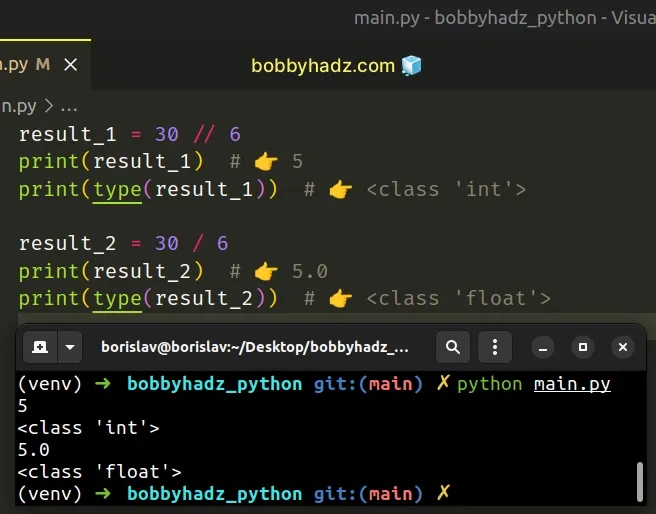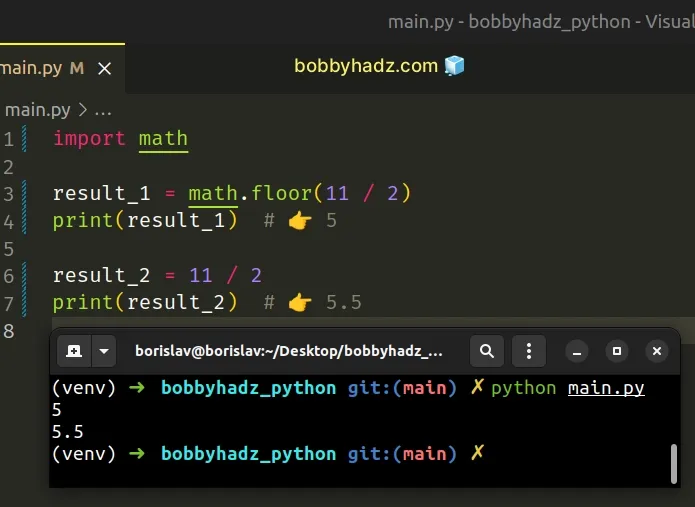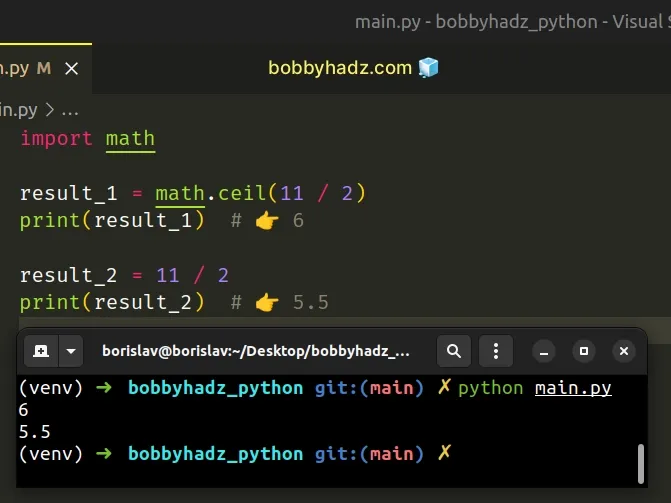# How to Divide without a remainder in PythonLast updated: Feb 20, 2023
2 min## #Divide without a remainder in Python

Use the floor division operator `//` to divide without a remainder.

The floor division operator will always return an integer and is like using mathematical division with the `floor()` function applied to the result.

main.py
```Copied!```result_1 = 30 // 6
print(result_1)  # 👉️ 5
print(type(result_1))  # 👉️ <class 'int'>

result_2 = 30 / 6
print(result_2)  # 👉️ 5.0
print(type(result_2))  # 👉️ <class 'float'>
``````We used the floor division `//` operator to return an integer from integer division.

Division `/` of integers yields a float, while floor division `//` of integers result in an integer.

The result of using the floor division operator is that of a mathematical division with the `floor()` function applied to the result.

main.py
```Copied!```print(11 // 2)  # 👉️ 5

print(11 / 2)  # 👉️ 5.5
``````

## #Using math.floor()

You can use the math.floor() method in a similar way to how we used the floor division `//` operator.

main.py
```Copied!```import math

result_1 = math.floor(11 / 2)
print(result_1)  # 👉️ 5

result_2 = 11 / 2
print(result_2)  # 👉️ 5.5
``````The math.floor method returns the largest integer less than or equal to the provided number.

## #Using math.ceil()

There is also a `math.ceil()` method.

main.py
```Copied!```import math

result_1 = math.ceil(11 / 2)
print(result_1)  # 👉️ 6

result_2 = 11 / 2
print(result_2)  # 👉️ 5.5
``````The math.ceil method returns the smallest integer greater than or equal to the provided number.

## #Using round()

You can also use the `round()` function if you want to round to the nearest integer when dividing.

main.py
```Copied!```result_1 = round(15 / 4)
print(result_1)  # 👉️ 4

result_2 = 15 / 4
print(result_2)  # 👉️ 3.75
``````

The round function takes the following 2 parameters:

NameDescription
`number`the number to round to `ndigits` precision after the decimal
`ndigits`the number of digits after the decimal, the number should have after the operation (optional)

The `round` function returns the number rounded to `ndigits` precision after the decimal point.

If `ndigits` is omitted, the function returns the nearest integer.

## #Divide without a remainder using int()

You can also use the `int()` class to remove the division decimal.

The `int()` class truncates floating-point numbers toward zero, so it will return an `int` that represents the number without the decimal places.

main.py
```Copied!```result_1 = int(7 / 2)
print(result_1)  # 👉️ 3

result_2 = int(-7 / 2)
print(result_2)  # 👉️ -3
``````

We used the `int()` class to remove the division decimal.

The int class returns an integer object constructed from the provided number or string argument.

If passed a floating-point number, the `int()` class truncates toward zero.

This approach allows us to remove the division decimal from a negative number and get a predictable result.

main.py
```Copied!```result_2 = int(-7 / 2) # 👉️ = -3.5
print(result_2)  # 👉️ -3
``````

## #Divide without a remainder using math.trunc()

Alternatively, you can use the `math.trunc()` method.

The `math.trunc()` method removes the fractional part and returns the integer part of the given number.

main.py
```Copied!```import math

result_1 = math.trunc(7 / 2)
print(result_1)  # 👉️ 3

result_2 = math.trunc(-7 / 2)
print(result_2)  # 👉️ -3
``````

The math.trunc method takes a number, removes its fractional part and returns its integer part.

The `math.trunc()` method rounds towards zero.

main.py
```Copied!```print(math.trunc(3.45))  # 👉️ 3

print(math.trunc(-3.45))  # 👉️ -3
``````

This approach achieves the same result as passing the result from the division to the `int()` class.

I wrote a book in which I share everything I know about how to become a better, more efficient programmer.You can use the search field on my Home Page to filter through all of my articles.